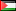##### Generalization of Faraday’s Law of Induction: Some Examples
Publication Type
Conference Paper
Authors
Fulltext

A general form of the induced electromotive force due to time-varying magnetic field is derived.
It is shown that the integral form of Faraday’s law of induction is more conveniently written in
the covering space. The method used in this work relies of finding the modified magnetic field
each time the circular path is traversed. This amounts to an additional time derivative of the
magnetic field. Therefore, the induced electromotive force comes from the sum of all
contributions coming from all winding numbers. Thus the differential form is shown to relate the
induced electric field in the
winding number to the

time- derivative of the
magnetic field. It is also shown that the higher order terms are modulated by the self-inductance
and resistance of the circuit. Some illustrative examples for time-dependent magnetic fields are
presented: Sinusoidal, exponential, and step-function fields. In each of these examples, it is
shown that the induced electromotive force could be written in closed analytical form that
depends (among other things) on the ratio between the self-inductance and resistance of the
circuit. Furthermore, in all these examples it is demonstrated that our result for the induced
electromotive reduces to the well-known result in the limit of the ratio of the self-inductance and
resistance goes to zero. The conclusion of this work shows that Maxwell’s equation of Faraday’s
law of induction can be written in a more general form in the physical space.

Conference
Conference Title
2015 Second International Conference on Physical Science and Technology
Conference Country
PalestineConference Date
Jan. 7, 2015 - Jan. 7, 2015